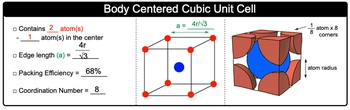## General Chemistry

Learn the toughest concepts covered in Chemistry with step-by-step video tutorials and practice problems by world-class tutors

13. Liquids, Solids & Intermolecular Forces

# Body Centered Cubic Unit Cell

Body Centered Cubic Unit Cell contains 2 atoms total.

Body Centered Cubic Unit Cell
1
concept

## Simple Cubic Unit Cell Concept 11m
Play a video:
the body center cubic unit cell is composed of a cube with an atom at each corner and one atom in the center. So we would say that when it comes to a body centered cubic unit some we have a total of two atoms involved. And remember one of those atoms is found in the center of our structure. Now remember each one of these red spheres. It is shared by other unit cells around. So each one of these only accounts for 1/8 of an atom. And if you took them all into consideration 1/8 times eight corners gives us one atom here. And then we have this one atom in the very center. So that's how we come up with two total atoms for body center, cubic unit self. Now we can say here that when it comes to a simple cubic unit cell we knew that the M. D. A. Or an edge length was equal to two R. That's because the atoms touched one another. But when it comes to a body centered cubic in itself there's a small little gap here as a result of this small gap. That means our edge link now changes our edge link now is equal to four times your radius divided by the square root of three. So a here equals four are divided by square root of three. Now, as we become more and more complex are packing efficiency will increase here for body centre cubic unit cell it becomes now 68%. And as we become more complex, our coordination number also increases here for body centered cubic unit cell, it now becomes the value of eight. So when dealing with body centered cubic unit cell, remember these key ideas and key values for any one of them.2
example

## Body Centered Cubic Unit Cell Example 140s
Play a video:
Iron adopts a body center, cubic units self. Illustrate how the number of atoms per unit cell for the iron atoms are obtained. So remember for any cubic unit, So we have 1/8 atom for every corner, and that's shared between eight atoms. So for all of them it's one atom right off the bat. But remember for a body centered cubic units. Now we also have an additional atom within the center of our cube, so we add that in. So taking those both into consideration, that's how we come up with two atoms per one unit cell for a body centered cubic unit self.
3
Problem

Thallium (I) iodide crystallizes with the same structure as CsCl, a compound with a body-centered cubic structure. The edge length of the unit cell of Tl is 4.20 angstroms. Calculate the ionic radius of Tl+. The ionic radius of I– is 2.16 angstroms.

4
Problem

Barium has a body-centered cubic structure. If the atomic radius of barium is 222 pm, calculate the density of solid barium.

5
Problem

The edge of a body-centered cubic unit cell of an element Y was found to be 3.16×10-8 cm. The density of the element is 19.35 g/cm3. What is the approximate molar mass of Y?